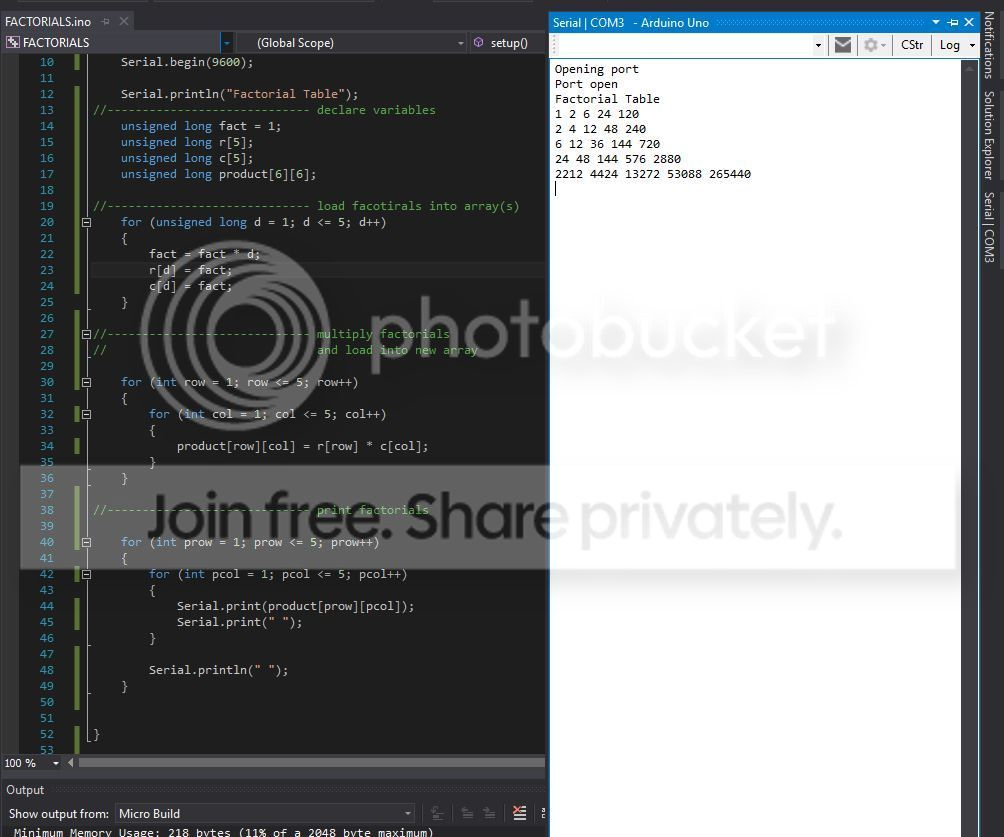# Multiplying Arrays

I have an issuing multiplying two one dimension arrays together. Each array is an unsigned long that is storing values of factorials. The last row is completely off. My code is below also with a screenshot of the output.

``````void setup() {
//----------------------------- start serial monitor
Serial.begin(9600);

Serial.println("Factorial Table");
//----------------------------- declare variables
unsigned long fact = 1;
unsigned long r;
unsigned long c;
unsigned long product;
for (unsigned long d = 1; d <= 5; d++)
{
fact = fact * d;
r[d] = fact;
c[d] = fact;
}
//----------------------------- multiply factorials
//                              and load into new array

for (int row = 1; row <= 5; row++)
{
for (int col = 1; col <= 5; col++)
{
product[row][col] = r[row] * c[col];
}
}

//----------------------------- print factorials

for (int prow = 1; prow <= 5; prow++)
{
for (int pcol = 1; pcol <= 5; pcol++)
{
Serial.print(product[prow][pcol]);
Serial.print(" ");
}

Serial.println(" ");
}
``````

}In C and its progeny, array indices start at 0. `unsigned long r` declares an array of five longs; the array elements are `r` through `r`. Your loop, however, varies the array index from 1 though 5. `r` is accessing whatever happens to lie in memory beyond `r`

Here is a much better version of your code. Note, among other things, the coding style. Remember that you write programs for other humans to read!

``````const int kSize = 5;
void setup() {
unsigned long r[kSize];
unsigned long product[kSize][kSize];
unsigned long fact = 1;
for (int d = 0; d < 5; ++d) {
fact *= d + 1;
r[d] = fact;
}
for (int row = 0; row < 5; ++row) {
for (int col = 0; col < 5; ++col) {
product[row][col] = r[row] * r[col];
}
}

Serial.begin(9600);
Serial.println("Factorial Table");
for (int row = 0; row < 5; ++row) {
for (int col = 0; col < 5; ++col) {
Serial.print(product[row][col]);
}
Serial.println();
}
}
``````

See the difference? Style points to note:

• No unnecessary space.
• The array size is used in several places; it's better to define it as a constant. There is also no reason to specify anything other than `int`; a good optimizer will detect that you are never using values of more than 5, and will optimize the code accordingly.
• You don't really need two separate arrays, since they have the same values.
• You obviously don't need to first store and then print, but I assume you are doing this to learn.
• I didn't initialize the Serial port and didn't print the header until i was ready to print. Group logically-related statements together. (OTOH, you might have been thinking that at some point you might want to insert print statements before there, so putting Serial.begin first thing in your code might have been better.

While there are lots of styles around, https://google.github.io/styleguide/cppguide.html is good enough for Google, so it's good enough for me :)

Here is a better way to initialize the array of factorials:

``````  r = 1;
for (int d = 1; d < kSize; ++d) {
r[d] = r[d - 1] * (d + 1);
}
``````
• Thank you for the help on the code. You made good suggestions. I didnt know I could use the same array for multiplying. Also I used an unsigned long in one of the for loops because I was getting weird numbers when calculating the factorials. The code you presented was very neat. Thank you also for the style guide link. – Michael Niebauer May 8 '16 at 16:27